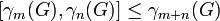# Second half of lower central series of nilpotent group comprises abelian groups

## Statement

Suppose$G$ is a nilpotent group of nilpotency class$c$. Define the lower central series of$G$ as follows:$\gamma_1(G) = G, \qquad \gamma_{m+1}(G) = [\gamma_m(G),G]$.

Then, for$k \ge (c + 1)/2$,$\gamma_k(G)$ is an abelian group, In particular,$\gamma_k(G)$ is an abelian characteristic subgroup.

## Facts used

1. Lower central series is strongly central: This states that$[\gamma_m(G),\gamma_n(G)] \le \gamma_{m+n}(G)$.

### Breakdown for upper central series

The first half of the upper central series of a nilpotent group need not comprise Abelian groups. In fact, even the second term of the series need not be Abelian, however large the nilpotence class. More specifically:

• Upper central series may be tight with respect to nilpotence class: For any natural number$c$, we can construct a nilpotent group such that the$k^{th}$ term of the upper central series of the group has nilpotence class precisely$k$ (note: the nilpotence class clearly cannot be greater than$k$, and this result says that tightness may hold.
• Second term of upper central series not is Abelian

## Proof

Given: A group$G$ of nilpotency class$c$.

To prove:$\gamma_k(G)$ is Abelian for$k \ge (c + 1)/2$.

Proof: By fact (1),$[\gamma_k(G), \gamma_k(G)] \le \gamma_{2k}(G) \le \gamma_{c+1}(G)$, and since$G$ has class$c$,$\gamma_{c+1}(G)$ is trivial. Thus,$[\gamma_k(G), \gamma_k(G)]$ is trivial, and thus,$\gamma_k(G)$ is an abelian group.